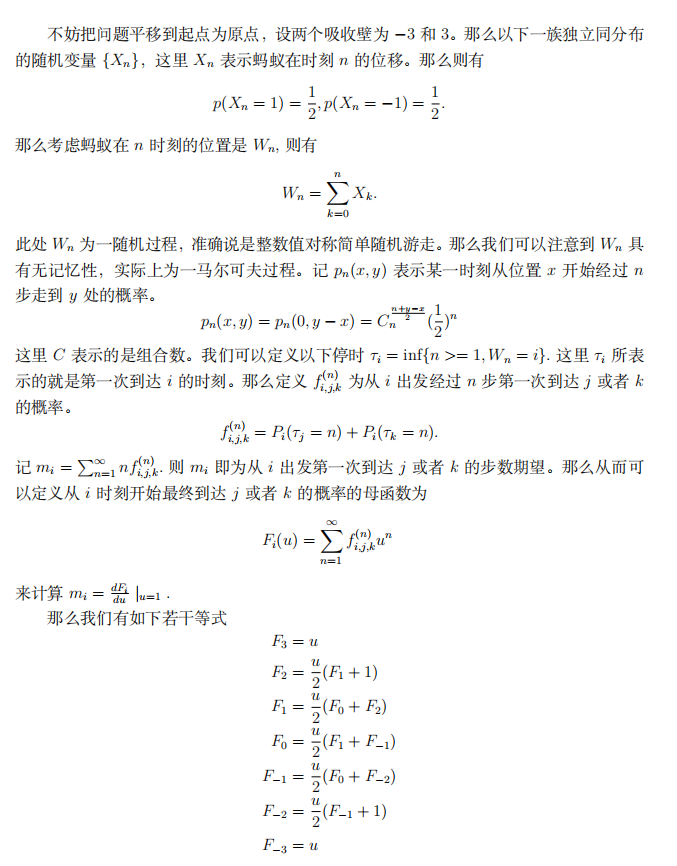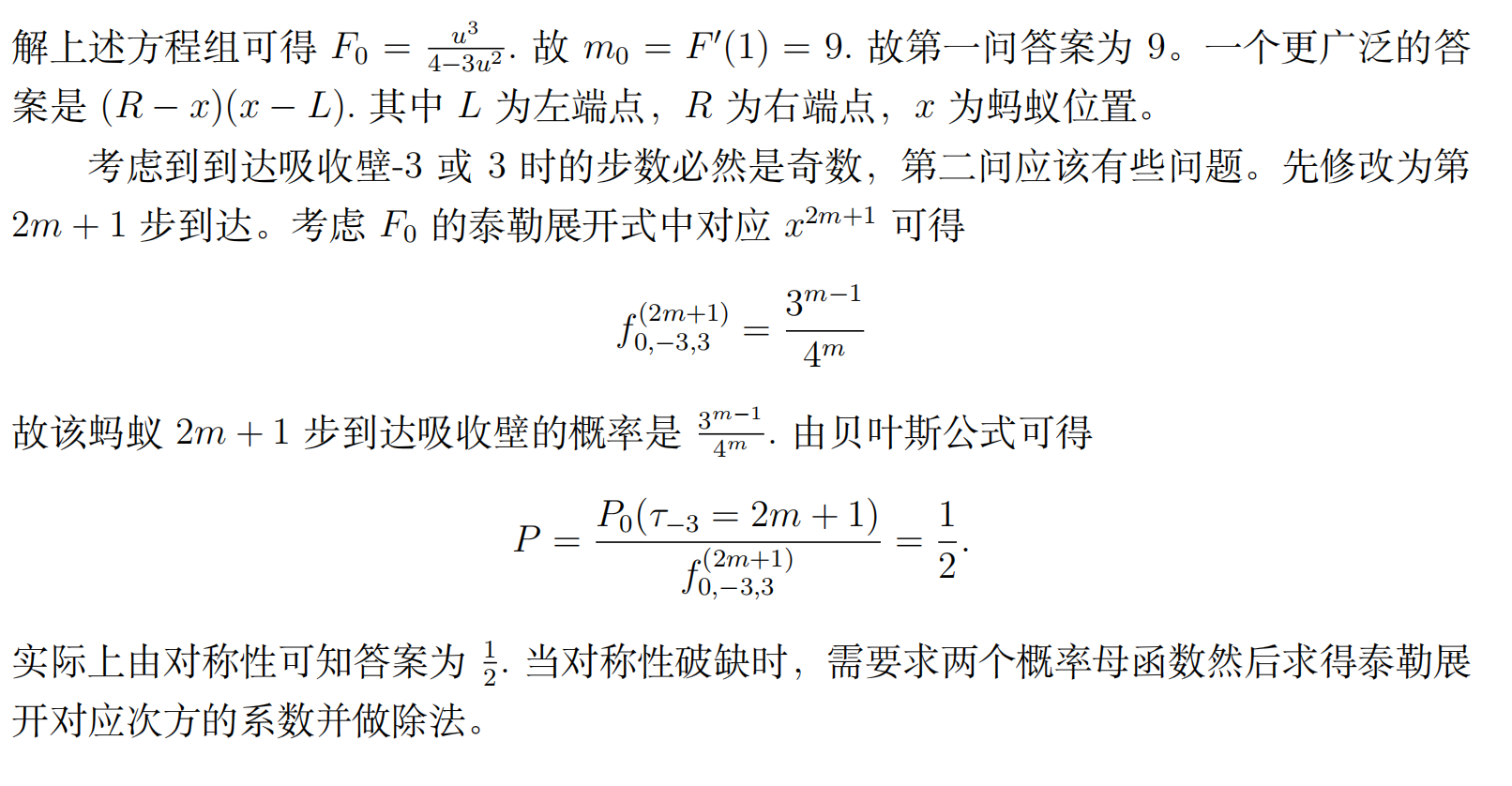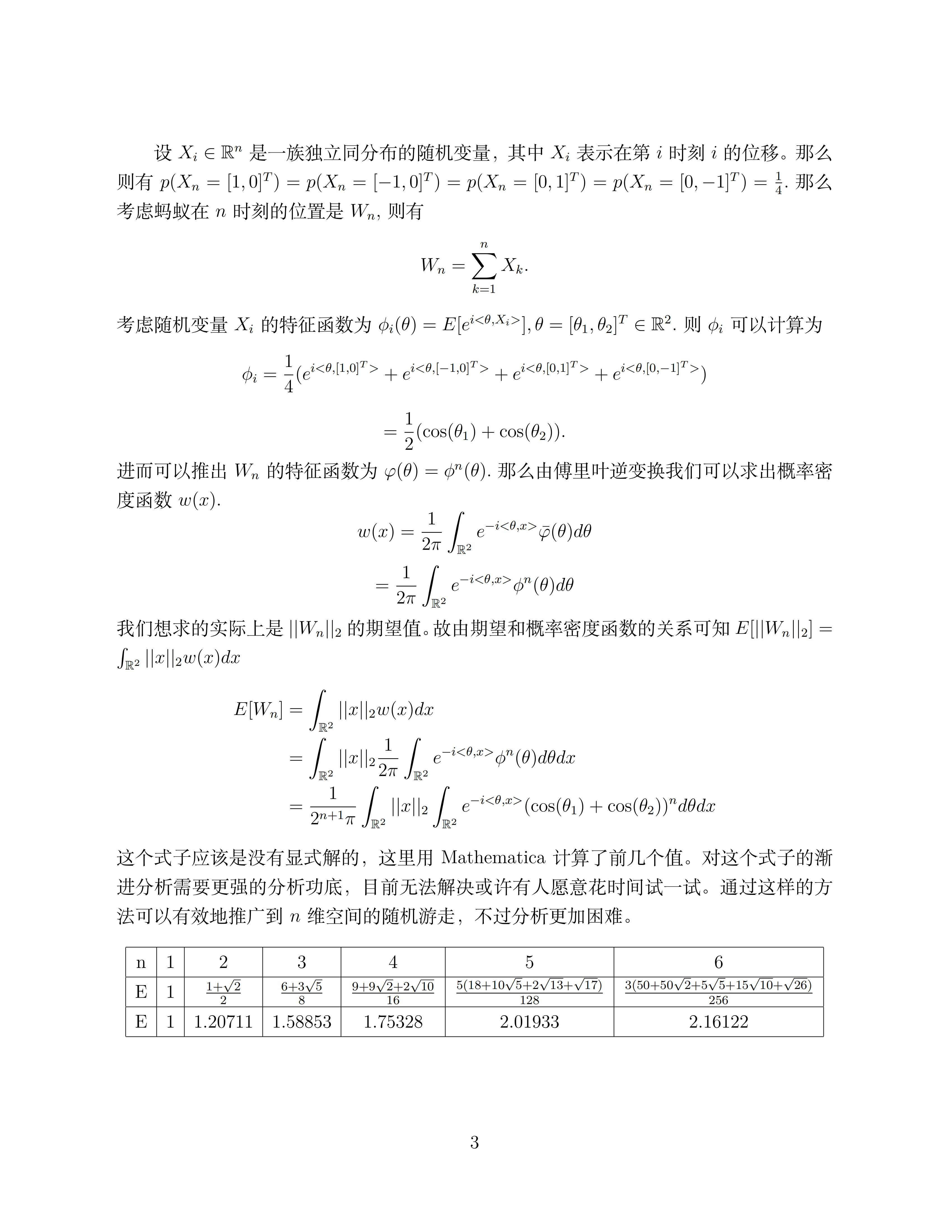# 解答

## 题目1

• n为偶：$n^n=(n^{(n/2)})^2$，n/2为整数，成立。[100:400]的任意偶数成立，一共有\$(400-100)/2+1=151个。
• n为奇：此时$n^n$为完全平方的条件是n为完全平方，因为$n^n = (x^2)^n = (x^n)^2$（设$n=x^2$）。所以$100 —> $10^2 —> $10 ，满足的奇数有11、13、15、17、19。

## 题目4

1. P(另一个是女孩|其中一个是女孩) = P(女女) / P(男女 or 女男 or 女女)=0.25/0.75=1/3

2. P(另一个是女孩|先出生的一个是女孩) = P(女女) / P(女男 or 女女)=0.25/0.5=0.5

3. P(另一个是女孩|有一个女儿叫维多利亚) = P(女女) / P(男女 or 女男 or 女女)=0.25/0.75=1/3

## 题目5

Brouwer fixed-point theorem（布劳威尔不动点定理）

1. 如果f(x)是连续的；
2. 且X为一非空的有界凸闭集(非空紧凸集)；
3. 则至少存在一个x*∈X使f(x*)=x*。即f(x)至少存在一个不动点。

## 题目6## 题目7$1--x->1; \ \ 1--y->2; \\ 2--z->1; \ \ 2--w->1 \\$

min. 4x+3y+3z+2w \\ s.t.\left\{ \begin{aligned} \ \ x+y=40 \ \ \ \ \ \ \ \\ \ \ z+w=30 \ \ \ \ \ \ \ \\ \ \ x+z=20 \ \ \ \ \ \ \ \\ \ \ y+w=50 \ \ \ \ \ \ \ \\ \end{aligned} \right.

$min(5x+3y+3z+2w) = min(x+200)$

## 题目8## 题目9

1. $C_n^k*p^k*(1-p)^{(n-k)}$

2. $1-(1-p)^n$

3. 掷硬币抛掷为独立事件，因此之前的抛掷情况不影响之后投掷结果的概率，因此答案是p。也可以用贝叶斯公式算一下。